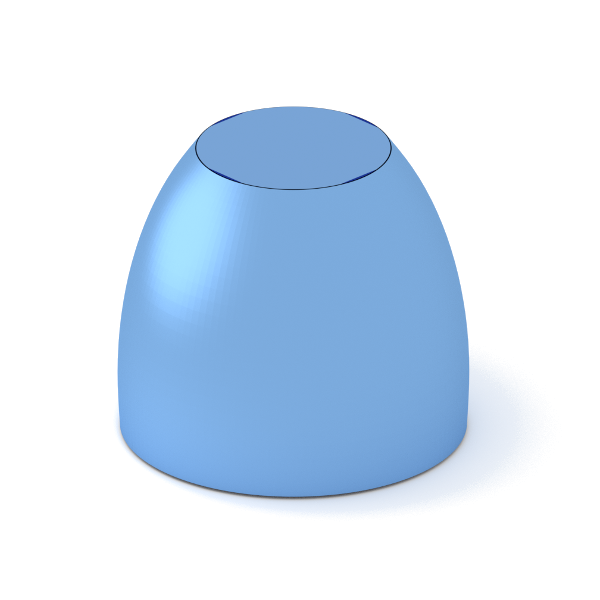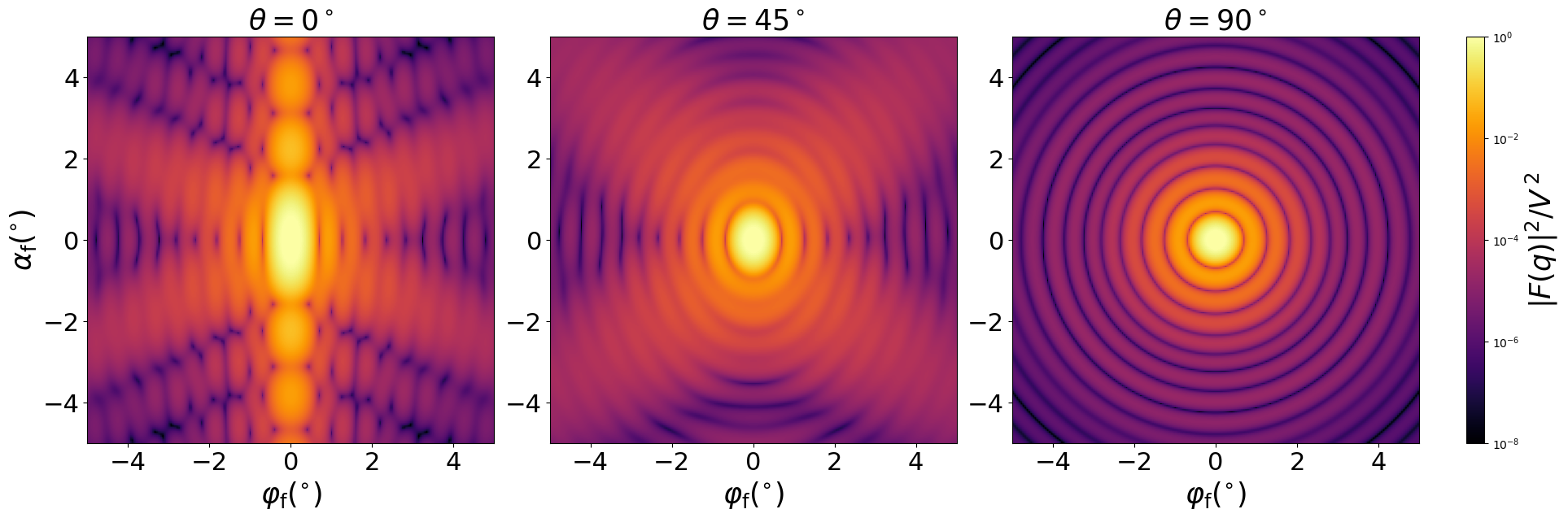### TruncatedSpheroid

A vertically oriented, horizontally truncated spheroid.#### Constructor

TruncatedSpheroid(R, H, f_p, dh)


Parameters:

• H, untruncated height (height before top removal)
• f_p, height flattering $f_p$
• dh, top removal

Constraint:

$dh < H \le 2f_pR$

#### Usage

As for any other Form factor.

#### Implementation

Class TruncatedSpheroid inherits from the interface class IFormFactor .

Computation involves numerical integration in vertical direction,

$$F(\mathbf{q})=2\pi \exp[iq_z(H-f_pR)] \int_{f_pR-H}^{f_pR-dh} \text{d}z \space R_z^2 \frac{J_1(q_{||}R_z)}{q_{||}R_z} \exp(iq_z z),$$

with the notation

$$q_{||} := \sqrt{q_x^2 + q_y^2}, \quad R_z:=\sqrt{R^2-z^2/f_p^2}$$

Volume has been validated against $$V=\dfrac{\pi}{3f_p^2} [ 3f_pR(H^2-dh^2) + dh^3 -H^3 ].$$

More special:

#### Example

Scattering by uncorrelated, oriented truncated spheroids for horizontal incidence. Rotation around $y$ axis:Generated by Examples/ff/TruncatedSpheroid.py .

#### History

Agrees with the IsGISAXS form factor “Spheroid” [manual, Eq. 2.42].